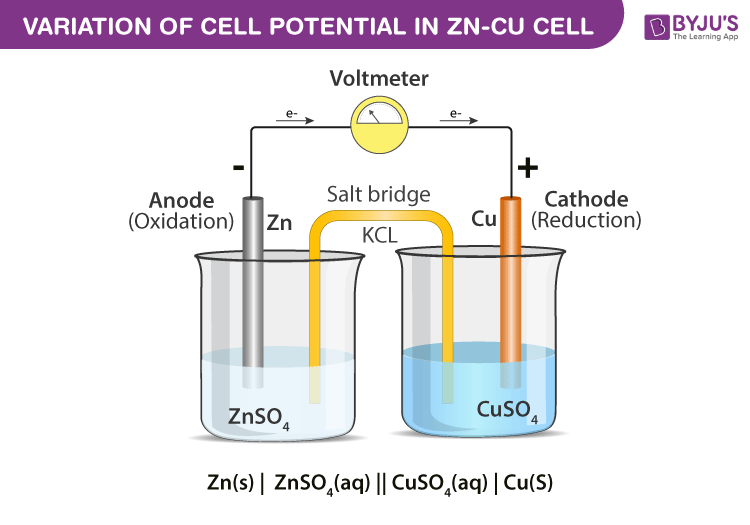# Variation of Cell Potential in Zn-Cu Cell

The potential difference between two galvanic cell electrodes is referred to as cell potential and is measured in volts. It is the difference between the cathode and anode’s potential for reduction or oxidation. It is called the cell’s electromotive force when no current is drawn from the cell.

## Aim:

To study the variation of cell potential in Zn/Zn2+ || Cu2+/Cu with change in concentration of electrolytes (CuSO4 or ZnSO4) at room temperature.

## Theory:

Electrochemistry deals with the interconversion of other forms of energy into electrical energy or vice versa. Potential difference is called cell potential or emf of the cell between the two electrodes. It is always measured in volts.

The reaction between copper sulfate solution and zinc metal forms the basis of Daniel cell. When this reaction is carried out in a test tube then no electricity is produced. If the same reaction is carried out in Daniel cell electricity is produced.

• Electrochemical reaction – The chemical reaction which takes place in a cell like Daniel cell.
• Electrochemical cell – Electrochemical reaction carried out in a specially designed set up.
• Electrolytic cells – Electrical energy is consumed to bring about a chemical reaction or a chemical change.
• Galvanic cells – Also called voltaic cells where chemical energy is converted into electrical energy.

In daniel cell current flows from copper electrode to the zinc electrode. Under Standard condition, it generates an emf of 1.1V.

The reaction taking place in Daniel cell are

Zinc electrode: Zn(s) → Zn2+(aq) + 2e

Copper electrode: Cu2+(aq) + 2e → Cu(s)

———————————————————————————————

Net reaction: Zn(s) + Cu2+(aq) → Zn2+(aq) + Cu(s)

———————————————————————————————

→ When Emf < 1.1V

Electrons flow from Zn rod to Cu rod and hence current flows from Cu to Zn electrode. Zinc dissolves at anode and copper deposits at cathode.

→ When Emf = 1.1V

The reaction stops altogether and there is no flow of electrons or current in the circuit. There is no chemical reactions at the electrode.

→ When Emf > 1.1V

When the current flows in opposite direction and the reaction proceeds in the opposite direction. Electrons flow from Cu to Zn and current flows from Zn to Cu.

## Materials Required:

1. 1M zinc sulfate solution
2. Copper sulfate solution
3. Zinc electrode
4. copper electrode
5. Beakers
6. Voltmeter (or) Potentiometer
7. Salt bridge
8. Connecting wires

## Apparatus Setup:## Procedure:

### Preparation of Salt Bridge:

• In a U-shaped tube heat 20gm of agar-agar gel and 5gm of potassium chloride in a beaker.
• Suck the molten mixtures into U-shaped tube.
• Allow it to cool.
1. Prepare 1M of zinc sulfate solution and 1M of copper sulfate solution in separate beakers.
2. Connect a salt bridge in such a manner that its one end dips in zinc sulfate and the other dips in copper sulfate solution.
3. Now connect the zinc plate to negative terminal of voltmeter and copper plate to positive terminal of voltmeter.
4. Note down the reading of voltmeter in observation table.
5. Take 10ml of 1M zinc sulfate and add water so as to make its volume up to 100ml. The resultant molarity will be 0.1M.
6. Now dip zinc rod in 0.1M zinc sulfate solution and copper rod in 1M copper sulfate solution and note the readings.
7. Repeat the same for 0.1M and 0.01M of zinc sulfate solution.
8. Note down the reading in voltmeter.
9. Repeat this procedure for other solutions of copper sulfate solutions.
10. Record electrode potentials values of copper electrodes for different concentrations.
11. Plot a graph for the variation of cell potential with concentration.
12. Calculate Ecell in each case and write down in the observation table.

## Observation and Inference:

 S.No [Cu2+(aq)]/mol L-1 log[Cu2+(aq)]/mol L-1 Ecell/V E(Cu2+/Cu) Experimental value 1 0.2 2 0.1 3 0.05 4 0.025 5 0.0125

## Calculations:

ECell = EoCell – log

EoCell = Eo(cathode) – Eo(anode)

R = 8.314

T = 298K

F = 96500C

n = 2 (number of electrons lost or gained)

By substituting all the values

ECell = EoCell – log

We can measure ECell at different concentration of electrolyte

## Results and Discussions:

1. The Ecell decreases with increase in molar concentration of [Zn2+]. (Zinc sulfate solution)
2. The Ecell increases with increase in molar concentration of [Cu2+]. (Copper sulfate solution)

## Precautions:

1. Immediately after use, place the salt bridge in distilled water.
2. Very carefully dilute the solution to another concentration.
3. Always clean strips of copper and zinc with sandpaper and connect the wires before use.
4. Choose appropriate graph plot scales.

## Viva Questions:

1. What is electrochemical cell?

Ans: An electrochemical cell is a device producing electrical energy from a spontaneous redox reaction. This type of cell includes the cell named after Luigi Galvani and Alessandro Volta, which is galvanic or voltaic.

2. Mention the names of anode and cathode of a daniel cell?

Ans: The names of anode and cathode of a daniel cell is

Anode – Zinc electrode, Cathode – Copper electrode

3. What is salt bridge?

Ans: A laboratory device used to connect half-cells of a galvanic cell, a type of electrochemical cell, with oxidation and reduction. It maintains electrical neutrality within the internal circuit, preventing the cell’s reaction to equilibrium from running rapidly.

4. What is the direction of flow of electrons in an electrochemical cell?

Ans: Electrons flow through an external wire from the anode into the cathode. The Daniell cell is a common galvanic cell.

5. Define the term EMF?

Ans: Electromotive force (emf) is an energy measurement that causes the flow of current through a circuit. It can also be defined as the possible charge difference between two points in a circuit. Also known as voltage, electromotive force is measured in volts.